# GSEB Class 8 Maths Notes Chapter 3 Understanding Quadrilaterals

This GSEB Class 8 Maths Notes Chapter 3 Understanding Quadrilaterals covers all the important topics and concepts as mentioned in the chapter.

## Understanding Quadrilaterals Class 8 GSEB Notes

→ Paper is an example of plane figure.

→ A plane figure formed by joining number of points without lifting pencil from the paper and without retracing any portion of the drawing other than single points is called a curve.

→ A curve which cuts itself is called a closed curve.

→ A simple closed curve made up of only line segments is called a polygon, e.g., triangle, rhombus, pentagon, hexagon, etc.

→ Convex polygons have no portion of their diagonals in their exteriors. This is not the case with concave polygons.

→ All the sides of a regular polygon have equal lengths, i.e., they are equilateral and equiangular.

→ Angle sum property: The sum of angles of a quadrilateral is 360°.

→ Diagonal is a line segment connecting two non-consecutive vertices of a polygon.

→ Number of diagonals in a polygon of n sides = $$\frac{n(n-3)}{2}$$

→ Sum of all exterior angles of a polygon = 360°.

1. Trapezium:
A quadrilateral having a pair of parallel sides is called a trapezium. Here, ABCD is a trapezium
In which $$\overline{\mathrm{AB}}$$ ∥ $$\overline{\mathrm{CD}}$$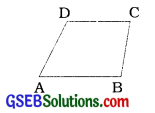2. Kite: A kite is a quadrilateral having two distinct consecutive pairs of sides of equal lengths. ABCD is a kite having AD = DC and AB=BC.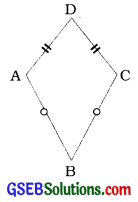3. Parallelogram:
A quadrilateral having its opposite sides parallel Is called a parallelogram.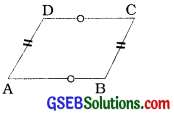In this figure ABCD is a parallelogram such that AB = CD and $$\overline{\mathrm{AB}}$$ ∥ $$\overline{\mathrm{CD}}$$
AD=BC and $$\overline{\mathrm{AD}}$$ ∥ $$\overline{\mathrm{BC}}$$Properties of parallelogram:

• The opposite sides of a parallelogram are parallel.
• The opposite sides of a parallelogram are equal.
• The opposite angles of a parallelogram are equal.
• The adjacent angles of a parallelogram are supplementary.
• The diagonals of a parallelogram bisect each other.
 Some Special Parallelogram Properties Rhombus: A parallelogram with all the sides of equal length.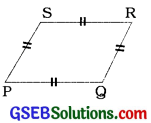1. Al the properties of a parallelogram. 2. DIagonals are perpendicular bisectors of each other. Rectangle: A parallelogram with all the right angles.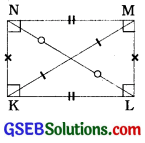1 All the properties of a parallelogram. 2. Each of the angles is a right angle. 3. DIagonals are equal. Square: A rectangle with all the sides of equal length.1. All the properties of a parallelogram. rhombus and a rectangle. 2. Each diagonal bisects the angle of the square. Kite: A quadrilateral with exactly two pairs of equal consecutive sides.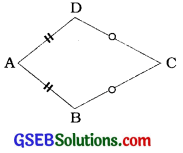1. The diagonals are perpendicular to each other. 2. One of the diagonals bisects the other. 3. In the figure m∠B=m∠D but m∠A ≠ m∠C. i.e., only one pair of opposite angles is equal.